# IntersectionSelection prerequisites: Two intersecting objects, which can be any of the following:

Two straight objects, circles, or arcs

One locus, function plot, or parametric plot and one straight object, circle, or arc

Two function plots on the same coordinate system

One polygon and one ray (command becomes First Intersection)

This Construct menu command constructs a point at each intersection of the two selected objects. If the objects actually intersect in multiple places, multiple points are constructed; otherwise one point is constructed.

The keyboard shortcut for Intersection is Shift+Ctrl+I (Windows) or Shift-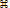I (Mac).

If you later drag the intersecting objects apart, the constructed intersection point disappears, and reappears when you drag the objects so that they’re again touching.

You can also construct a point of intersection by clicking the intersection with the Selection Arrow tool or with any tool that constructs points as part of its operation (such as the or Straightedge tools).

 Selected Objects: Resulting Construction: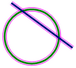One circle, one straight object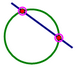Two intersections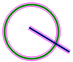One circle, one straight object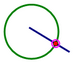One intersectionOne locus, one arc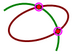Two intersectionsTwo function plotsThree intersectionsOne ray, one polygon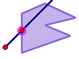First intersection